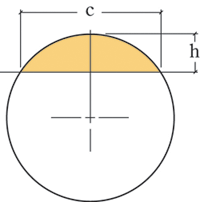# Calculating a Curve RadiusHow do I calculate the radius of a curve that will fit a particular height and width?

Michael Dresdner: The formula is r = (c2+4h2)/(8h) where r is the radius, c is the length of the cord, (the width across the terminating ends of the curve) and h is the height from the center of the cord to the top of the curve.